## RS Aggarwal Solutions Class 10 Chapter 9 Mean, Median, Mode of Grouped Data,Cumulative Frequency Graph and O give Ex 9e

These Solutions are part of RS Aggarwal Solutions Class 10. Here we have given RS Aggarwal Solutions Class 10 Chapter 9 Mean, Median, Mode of Grouped Data,Cumulative Frequency Graph and O give Ex 9e.

Other Exercises

Question 1.
Solution:We plot the points (10, 5), (20, 8), (30, 12), (40, 15), (50, 18), (60, 22), (70, 29), (80, 38), (90, 45) and (100, 53) to get the ‘less than type’ ogive as follows: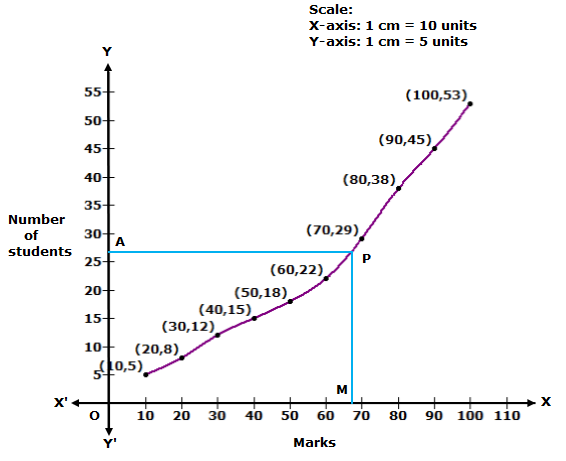Here, N = 53 ⇒ N/2 = 26.5.
At y = 26.5, affix A.
Through A, draw a horizontal line meeting the curve at P.
Through P, a vertical line is drawn which meets OX at M.
OM = 68 units
Hence, median marks = 68

Question 2.
Solution:
We plot the points (15, 2), (30, 5), (45, 9), (60, 17), (75, 39), (90, 54), (105, 70) and (120, 80) to get the ‘less than type’ ogive as follows: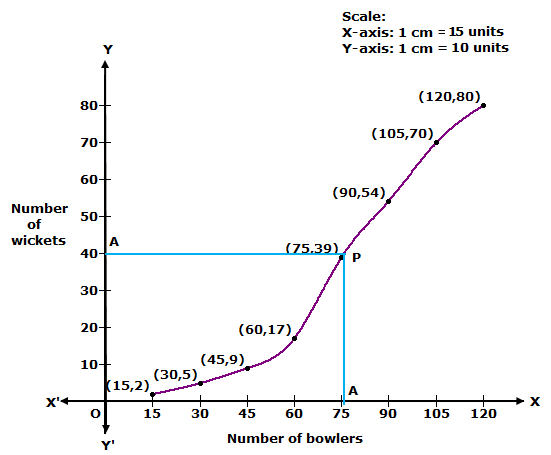Here, N = 80 ⇒ N/2 = 40.
At y = 40, affix A.
Through A, draw a horizontal line meeting the curve at P.
Through P, a vertical line is drawn which meets OX at M.
OM = 78 units
Hence, median number of wickets = 78

Question 3.
Solution:We plot the points (0, 100), (10, 96), (20, 90), (30, 80), (40, 70), (50, 45), (60, 23) and (70, 5) to get the ‘more than type’ ogive as follows: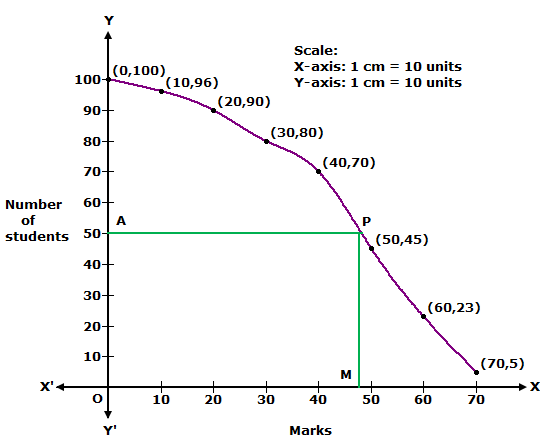Here, N = 100 ⇒ N/2 = 50.
At y = 50, affix A.
Through A, draw a horizontal line meeting the curve at P.
Through P, a vertical line is drawn which meets OX at M.
OM = 47 units
Hence, median marks = 47.

Question 4.
Solution:We plot the points (135, 50), (140, 45), (145, 37), (150, 28), (155, 16) and (160, 2) to get the ‘more than type’ ogive as follows: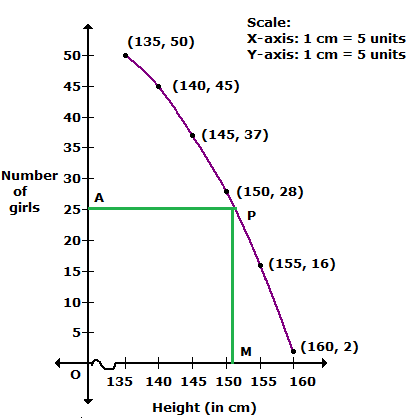Here, N = 50 ⇒ N/2 = 25.
At y = 25, affix A.
Through A, draw a horizontal line meeting the curve at P.
Through P, a vertical line is drawn which meets OX at M.
OM = 151 units
Hence, median height = 151 cm.

Question 5.
Solution:We plot the points (140, 156), (160, 153), (180, 145), (200, 130), (220, 90), (240, 40) and (260, 10) to get the ‘more than type’ ogive as follows:Here, N = 156 ⇒ N/2 = 78.
At y = 78, affix A.
Through A, draw a horizontal line meeting the curve at P.
Through P, a vertical line is drawn which meets OX at M.
OM = 226 units
Hence, median consumption of electricity = 226 units

Question 6.
Solution:We plot the points (50, 100), (55, 98), (60, 90), (65, 78), (70, 54)and (75, 16) to get the ‘more than type’ ogive as follows:Here, N = 100 ⇒ N/2 = 50.
At y = 50, affix A.
Through A, draw a horizontal line meeting the curve at P.
Through P, a vertical line is drawn which meets OX at M.
OM = 70.5 units
Hence, median production yield = 70.5 kg/ha

Question 7.
Solution:
Less Than Series:

 Class interval Cumulative Frequency Less than 200 5 Less than 300 11 Less than 400 22 Less than 500 35 Less than 600 40 Less than 700 44 Less than 800 47 Less than 900 49

We plot the points (200, 5), (300, 11), (400, 22), (500, 35), (600, 40), (700, 44), (800, 47) and (900, 49) to get ‘less than type’ ogive.

More Than Series:

 Class interval Frequency More than 100 49 More than 200 44 More than 300 38 More than 400 27 More than 500 14 More than 600 9 More than 700 5 More than 800 2

We plot the points (100, 49), (200, 44), (300, 38), (400, 27), (500, 14), (600,9), (700, 5) and (800, 2) to get more than ogive.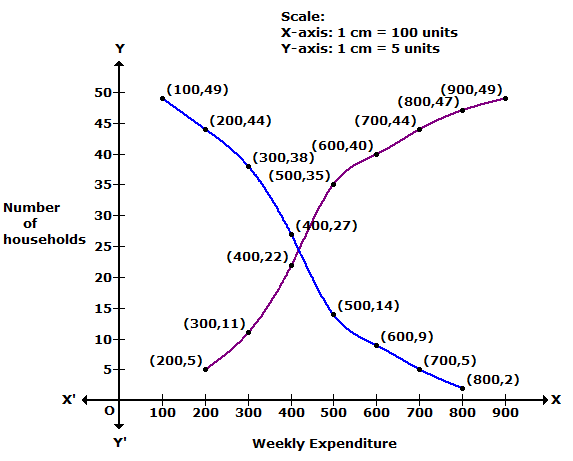Question 8.
Solution:
More than series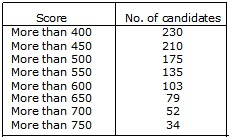We plot the points (400, 230), (450, 210), (500, 175), (550, 135), (600, 103), (650, 79), (700, 52), (750, 34)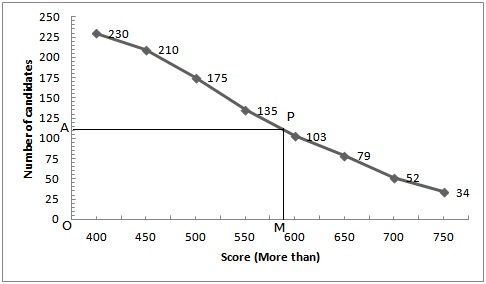Hence,N = 230 ⇒ N/2 = 115.
Take a point A(0, 115) on the y-axis and draw AP||x-axis meeting the curve at P, Draw PM ⊥ x-axis intersecting x-axis at M
Then,OM = 590
Hence median = 590.

Question 9.
Solution:
(i) Less than series: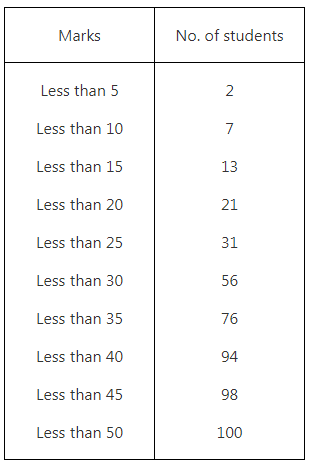Plot the points (5, 2), (10, 7), (15, 13), (20, 21), (25, 31), (30, 56), (35, 76), (40, 94), (45, 98) and (50, 100)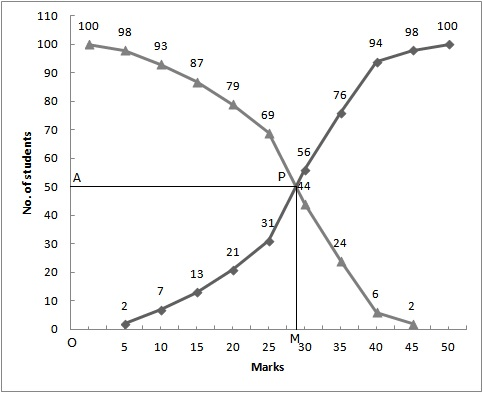Join these points free hand to get the curve representing “less than” cumulative curve.

(ii)From the given table we may prepare the ‘more than’ series as shown below:Now, on the same graph paper as above, we plot the point (0, 100), (5, 98), (10, 93), (15, 87), (20, 79), (25, 69), (30, 44), (35, 24) and (40, 6) and (45, 2)
Join these points free hand to get required curve
Here N = 100 ⇒ N/2 = 50
Two curves intersect at point P(28, 50)
Hence, the median = 28.

Question 10.
Solution:
We may prepare less than series and more than series

(i)Less than series :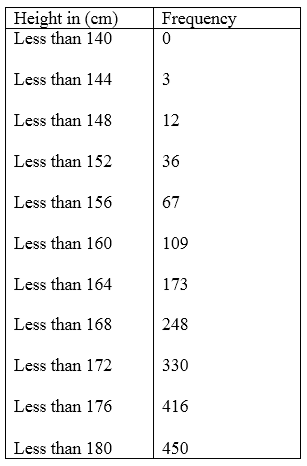Now on graph paper plot the points (140, 0), (144, 3), (148, 12), (152, 36), (156, 67), (160, 109), (164, 173), (168, 248), (172, 330), (176, 416), (180, 450)

(ii)More than series :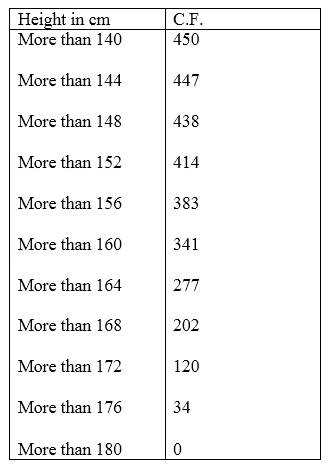Now on the same graph plot the points (140, 450), (144, 447), (148, 438), (152, 414), (156, 383), (160, 341), (164, 277), (168, 202), (172, 120), (176, 34), (180, 0)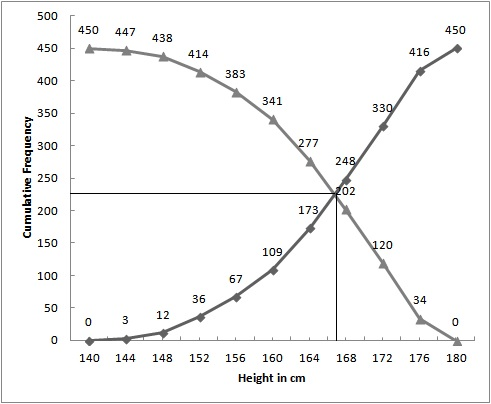The curves intersect at (167, 225).
Hence, 167 is the median.

We hope the RS Aggarwal Solutions Class 10 Chapter 9 Mean, Median, Mode of Grouped Data Ex 9e help you. If you have any query regarding RS Aggarwal Solutions Class 10 Chapter 9 Mean, Median, Mode of Grouped Data Ex 9e, drop a comment below and we will get back to you at the earliest.